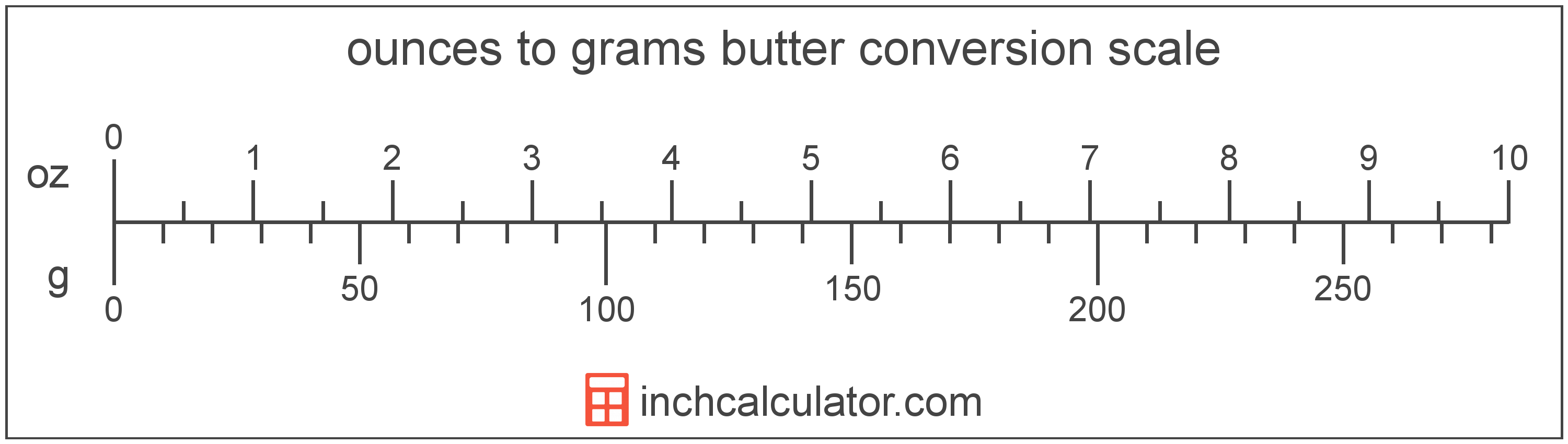# Convert Ounces of Butter to Grams

Enter the butter in ounces below to get the value converted to grams.

Results in Grams:1 oz = 28.3496 g

Do you want to convert grams to ounces?

## How to Convert Ounces to Grams

To convert a measurement in ounces to a measurement in grams, multiply the butter by the following conversion ratio: 28.3496 grams/ounce.

Since one ounce of butter is equal to 28.3496 grams, you can use this simple formula to convert:

grams = ounces × 28.3496

The butter in grams is equal to the butter in ounces multiplied by 28.3496.

For example, here's how to convert 5 ounces to grams using the formula above.
grams = (5 oz × 28.3496) = 141.748 g### How Many Grams Are in an Ounce of Butter?

There are 28.3496 grams in an ounce of butter, which is why we use this value in the formula above.

1 oz = 28.3496 g

## What is an Ounce?

In the US butter is sold in 16 ounce packages, which contain 1 pound of butter.

The ounce is a US customary and imperial unit of weight. Ounces can be abbreviated as oz; for example, 1 ounce can be written as 1 oz.

## What is a Gram?

Butter has a density of 911 grams per liter, and one stick of butter weighs about 113.4 grams.

The gram, or gramme, is an SI unit of weight in the metric system. Grams can be abbreviated as g; for example, 1 gram can be written as 1 g.

## Ounce to Gram Conversion Table

Table showing various ounce measurements converted to grams.
Ounces Grams
1 oz 28.35 g
2 oz 56.7 g
3 oz 85.05 g
4 oz 113.4 g
5 oz 141.75 g
6 oz 170.1 g
7 oz 198.45 g
8 oz 226.8 g
9 oz 255.15 g
10 oz 283.5 g
11 oz 311.85 g
12 oz 340.2 g
13 oz 368.54 g
14 oz 396.89 g
15 oz 425.24 g
16 oz 453.59 g
17 oz 481.94 g
18 oz 510.29 g
19 oz 538.64 g
20 oz 566.99 g
21 oz 595.34 g
22 oz 623.69 g
23 oz 652.04 g
24 oz 680.39 g
25 oz 708.74 g
26 oz 737.09 g
27 oz 765.44 g
28 oz 793.79 g
29 oz 822.14 g
30 oz 850.49 g
31 oz 878.84 g
32 oz 907.19 g
33 oz 935.54 g
34 oz 963.89 g
35 oz 992.24 g
36 oz 1,021 g
37 oz 1,049 g
38 oz 1,077 g
39 oz 1,106 g
40 oz 1,134 g